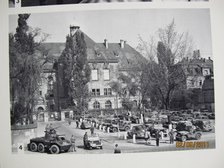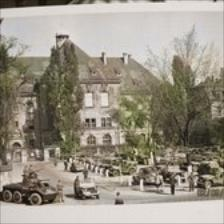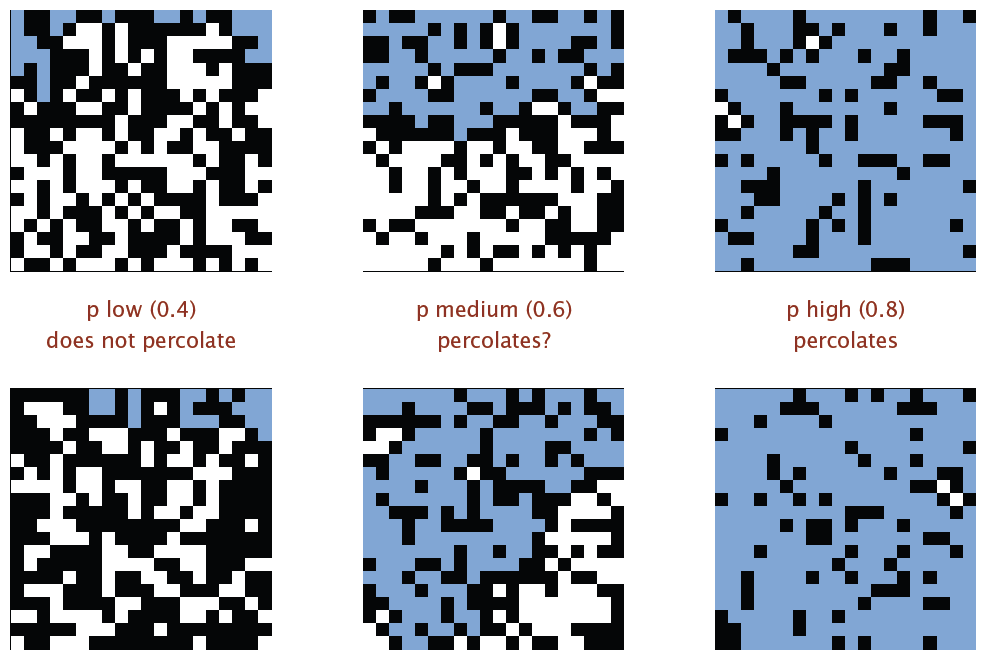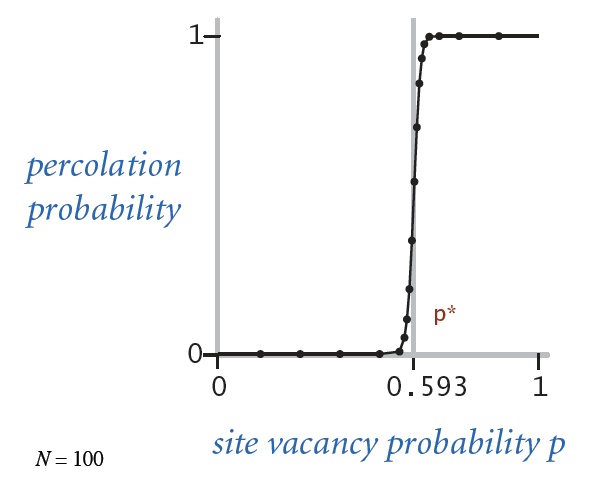# A gentle overview of the pipeline in Protein Structure Prediction

Disclaimer: the author has a background in Computer Science, the physics, chemistry and biology anecdotes in this article are obtained during research on the topic on the on-demand basis, without a formal training in the respective subjects. Also, this article aims to describe the pipeline to help the readers to form initial intuition about the problem and approach. Details of the machine learning part, is not within my intended scope of this article.

## ELI5 Protein Structure Prediction problem

Proteins are basic building blocks of life. They themselves are messy ball-like objects much similar to carelessly rolled up shoe-laces. A single shoe-lace is a string of amino acids. Amino Acids are even smaller stuff that are very important to life. Interestingly, groups of amino acids forms different shape under different conditions. They are like living lego blocks, some combination of these blocks tend to stick together, like magnets; and almost all of them act like this depending on whether they can relax themselves in water. We know that proteins can do a lot of different work, and we can tell what type of work a protein can do by looking at its shape. The shape of protein is like an outfit for job, to us humans: we can tell a person is a police officer because that person is wearing a police outfit. However, they are many many proteins, so the biology and chemistry experts documented them using a technique called “sequencing”: putting down each amino acid one by one: Imagine straightening a rolled shoe-lace magically without having to worry about knots. This technique is so fast, it allows us to document massive amount of proteins, but at the same time we lose the shape of the protein. As a result, only a small part of the known proteins have their shape mapped out. Finding out the rolled up shape base only on the straightened shoe-lace is what we called “Protein structure prediction problem”.

How hard can it be? As it turns out, quite complicated :‘(Above is an illustration of broken down steps of how we predict a folded protein: as you can see, getting the final structure or even the tertiary structure right involves a lot of hidden knowledge from physics, chemistry and biology.

## Hold your breath: Terminology and Structural Physics of the Protein

Firstly, we will use abbreviation AA for amino acids. The magnet that binds AA is called peptide bonds. We sometimes also say conformation (of AA sub-sequence or the whole chain), instead of the shape.

The bonds bind a small sequence of AA, different ordering and different type of AA forms different local shapes. We call these local shapes Secondary Structures(SS), they mostly fall into 2 groups: $\alpha$-helix and $\beta$-sheet. Also, SS generally have a certain range of length for their respective type. $\alpha$-helix is usually 3 to 5 AA long, while $\beta$-sheet averagely could stretch to 7 AA long.

The conformation of “strings” of SS (Tertiary Structure) is mainly determined by compounded bond force of all the AA in the chain. On the even smaller scale, AA’s carbon atom ($C_{\alpha}$) serves as pivot point, on which the chain rotates: The positive and negative polar charge of each AA interact (attracts and propels) and define a “stable” conformation, depending on a series of rotating dynamics. To make matters even more “interesting”, given the same sub-sequence, some known conformation of the said sequence don’t end up in the same conformation in other part of the chain, because that part is not interacting with water.

To recap, the conformation of protein is important because the structure reveals the function of protein. Many people equate protein to machines, and this analogy is quite fitting: on the molecular level, a factory of proteins breaks up and assemble genes as if they are workers of different parts on the manufacturing line.

Without loss of generality, a rigorous physical rule-set describes the mechanics of proteins, thus in principle life itself can be understood, should we be able to gain full knowledge of the dynamics and their functional mappings.

## Methodology: a tale of two cites

Evidently due to the complexity of the problem, traditional approaches of structural prediction involve the comparison against known structures. The assumption is that the evolution has done enough experiments for us to say, if a stable and working structure happened before (“happened” as in proven, not extinct), it should happen again elsewhere. As a matter of fact, the comparison method is effective. The main problem, however, is that we don’t have nearly enough of known templates to solve the rest of protein sequences.

For sequences that have nearly zero matching templates, we have to seek solutions that assume almost nothing, that derive from first principles. This is what we (Computer Science, Computational Biology) call the ab initio or de novo methods.

de novo or ab initio is latin synonym for “from scratch”. Because we at least know about the physical bond properties and constraints, we should be able to compute based on energy (how likely, or least amount of energy, for a conformation to take hold).

From the early hand-crafted rule based simulations to the more recent machine learning approaches, ab initio methods continue to gain traction. For instance, SS prediction using bi-directional recurrent neural networks can achieve an accuracy of well beyond 80%, and each iteration seemed not have slowed down, continuing the approach to the theoretical limit of 90% accuracy.

So how does a pipeline for ab initio Protein Structure Prediction task looks like?

## Pipeline for ab inito or de novo Protein Structure Prediction

Machine learning is data-driven, more meaningful data is always better than less. Instead of taking sequences alone as input for training in a machine learning approach, we enrich them using a series of pre-processing steps. Although we don’t have templates to look-up from, we still can gain insight from sequence similarities. In Computational Biology we can gain richer protein profile by aligning sequences based on similarities. Protein sequences are coded sequences of AA. We have 20 known AA, thus we codify them using alphabet A-T. Similarity includes, but not limited to, alphabetical similarity of the sequence, sub-sequence AA substitution tables and similarity produced by probabilistic models. Commonly the main alignment and search methods are PSI-BLAST and HHblits. The resulting richer data-set is then further encoded and primed for training.

Mirroring the illustration in the first section, we design a multi-stage machine learning model: Predicting SS and other properties first, using predicted SS feature and original profile to predict distance/contact maps of the tertiary features, eventually combining all previous information to predict final folded structure.

For those readers who reach here and find this text helpful, I thank you for your attention. And I hope you to talk about the details of my approach in a later article soon.

# CNN Black-White Photo to Colour Photo Conversion

http://tinyclouds.org/colorize/# Using Go and Monte Carlo Method to obtain Percolation Threshold

This article is inspired by Slides in the Algorithms, Part I by Kevin Wayne, Robert Sedgewick. Image 1 and 2 are cropped from the original slide on page 51 and 52 of the 15UnionFind.PDF *

My knowledge is limited, my writing is most certainly flawed. Please don’t hesitate to leave me a feedback/suggestion/correction/rant

# Percolation and the threshold

In computer science, especially algorithm there is a topic that involves solving dynamic connectivity. Figuring out if a path/route is connected to other path/route is widely applicable in engineering the solutions to real-world problems.

For instance in many physical systems we have a model of N by N square grid, the blocks inside the grid can be either open or blocked. We call the system grid is percolated if and only if there is a path connecting top open sites with bottom open sites. Just like solving a labyrinth problem, on a massive grid it is very tiresome and ineffective (enumerate all possibility. Here checking whether two components are connected, a brute-force will cost $O(n^2)$ time) to obtain the percolating status. Therefore we need an efficient algorithm to solve this percolation problem. Determining whether 2 subset of the grid is connected can be modeled as connected components as in graph theory, which involves equivalence relation in the Set Theory:

On set X, an operation ~ is an equivalence relation iff a ~ a if a~b then b~a if a~b and b~c then a~c

Intuitively, subgraphs (components) are “equivalent” iff they have the same root. Because we don’t need to keep track of the shapes of the original subgraphs, we can model the solution to support a tree like data structure that is as flat as possible to guarantee the look-up speed, and some Connect (union) action to merge two subgraphs. In other words, we can choose Union Find algorithm to solve the percolation problem.

There is however a subsequent question regarding percolation that is more interesting: if all the grid blocks have a possibility p to be opened (blocked would be (1-p)), what is the likelihood that the whole system percolates? Imaging a social network with N by N user grid, each user has the possibility of p to be able to communicate with his/her neighbor, what are the odds that the first row users are linked with the bottom row users?We observed that above a certain p, the system most likely percolates. This critical threshold p is called Percolation Threshold. It appears to be a constant number, tied to the lattice structure.There is no direct solution to calculate the percolation threshold. But we sure can simulate the problem and if we do enough times of experiments, we will have some confidence that an approximation can be determined by the experiment results.

# Approach

Due to the fact that Simulations like Monte Carlo require iterating huge number of randomized experiments in order to deliver confident results, the number class we are talking here often start with millions. Running such simulations might be challenging if we have

1. Inefficient Experiments (software constraints)
2. Slow Computer (hardware constraints)

Point 1 might be overcome by using more efficient algorithms. However faster machines may not be available, especially since 2000 the trend of new computing engine is to go multi-core instead of higher frequency. This is why parallelism is often the way to go for such problems. I chose to use Go because on the one hand, it’s a fantastic system language which is fast, expressive and most familiar to C programmers; on the other hand, it has first class support for concurrency programming. Although the CSP paper by Hoare has been around for decades, there are very few of mainstream languages that adopt the concept.

# Concurrent Analysis

Each experiment in the simulation is independent to each other, this is a perfect entry-point where we can apply parallelism. Mainly we need to satisfy the condition

$$\begin{equation} P_{linear} (x) = P_{parallel} (x) \end{equation}$$
With
$$\begin{equation} P_{linear}(x) = \dfrac{\sum^n{p(x)}}{n} \end{equation}$$

where n is the number of all experiments, and $p(x)$ being the probability of experiment $x$ that yields a percolation.

And having denoted $w$ as the workload every worker has to perform and the $c$ is the count of all the works, the parallel version needs to deliver

$$\begin{equation} P_{parallel}(x) = \dfrac{\sum^c \sum^w{p(x)}}{n} \text{, with } w\cdot c = n \end{equation}$$

# The experiment

Ideally if we can have a grid constructed for us in a manner that it is instantly set up with randomized open sites, all we need to do is call the Find function on the grid (the $p(x)$ in previous section).

If I am to randomly fill up a completely blocked grid with open sites, it would take me maximal SIZE of the grid times to construct a percolation. Further, if I repeat the random construction again and again and record the steps that I took to produce a percolation in each and every experiment $\rightarrow$ when making a histogram of this recorded steps, I would have a plot that looks like a normal distribution which centers at the expected value. This expected value is exactly the wanted $p$ because the underlying pdf (probability density function) is what the plot represents.

To recap and modify our formula.

Let $s(x)$ be the steps used in $x$ experiment to produce a percolation; $n$ be the number of experiments; $g$ be the number of the blocks in the grid (size).

$$\begin{equation} P(x)=\dfrac{\sum^n{s(x)}}{n\cdot g} \end{equation}$$

Because $\dfrac{s(x)}{g} = p(x)$, we can parallel this approach on the top level too.

## High Level Algorithm

To randomly fill up open sites on a initially blocked square grid, is equivalent to obtaining a randomized permutation of numbers $0...N$-$1$, where $N$is the size of the grid. The rand package of the Go library can deliver that right out of the box.

On each site opening I need to connect the opened site to its neighbors, iff the neighbor is also opened.

Algorithm in Pseudo-code

Step := 0
LOOP number in Permutation
OBTAIN Neighbors of number
LOOP neighbor in Neighbors
IF neighbor is open
Connect / Union number with neighbor
Step++
IF Percolates / Find
RETURN Step
END IF
END IF
END LOOP
END LOOP


## Construction and Union Find

Now we need to construct the grid using cheap and smart data structures that supports our efficient union find algorithm. Let the size of the grid be $Size = N^2$, N is the length of its side. A weighted compressed Union-Find can achieve both union and find in $O(N+Mlg^*N)$time. * This Union-Find needs two arrays of size $N^2$, one (noted P) for presenting the parent relation / subgraph ownership of each element, the other (noted S) for the size of that component (used for weight comparison).

Additionally, in order to maintain states of the openness of the blocks, I need an boolean array of $N^2$. Finally, to ease the modeling of opening to top side edge and bottom side edge, we can add 2 virtual sites $N^2 + 1$ and $N^2 + 2$ to the original P array, the grid percolates iff

$$\begin{equation} N^2+1 \text{ and } N^2+2 \end{equation}$$
are connected.

# Code

Code contains 1 package called connectivity, which contains 2 components: unionfind and percolation. Both components are exported, you can use it on your own project. To run the simulation, please see next section.

Complete source code on Github

## Getting the code

go get github.com/nilbot/algo.go/connectivity



## Some Snippets

A Simulator interface:

type Simulator interface {
Simulate() int64
mark(n int)
Clear()
}


Data structure used in simulation

type PercolationSimulator struct {
Size    int
Marked  []bool
l       int
connect *WeightedCompressed
}


and its construction

func NewPercolationSimulator(N int) *PercolationSimulator {
result := &PercolationSimulator{
Size:    N * N,
Marked:  make([]bool, N*N),
l:       N,
connect: NewWeightedCompression(N*N + 2),
}
for i := 0; i != N; i++ {
result.connect.Union(i, result.Size)
}
for i := N * (N - 1); i != N*N; i++ {
result.connect.Union(i, result.Size+1)
}
return result
}


The 2 for loops are the trick to have 2 extra virtual sites connected to top and bottom edges, respectively.

To obtain a randomized permutation, calling (r *rand) Perm(int) from the math/rand package.

// return a slice of pseudo-random permutation of [0,n)
func getPermutation(n int) []int {
seed := time.Now().UnixNano() % 271828182833
r := rand.New(rand.NewSource(seed))
return r.Perm(n)
}


And marking open site, as outlined in pseudo-code in previous section:

// mark (paint) the block as white
func (p *PercolationSimulator) mark(n int) {
if p.Marked[n] {
return
}
p.Marked[n] = true
neighbors := getDirectNeighbours(n, p.l)
for _, adj := range neighbors {
}
}
}


For overview you can also visit the godoc

# Result and Benchmarks

## Run Test and Benchmark

cd \$GOPATH/github.com/nilbot/algo.go/connectivity
go test -bench Simulate


## Result

Benchmark

BenchmarkSimulate     100000         21375 ns/op


was achieved on a 2013 i7 8G MacbookAir. On the same machine running simulation with 10 million iterations yields

ran 1000000 simulations, got result 0.59377084